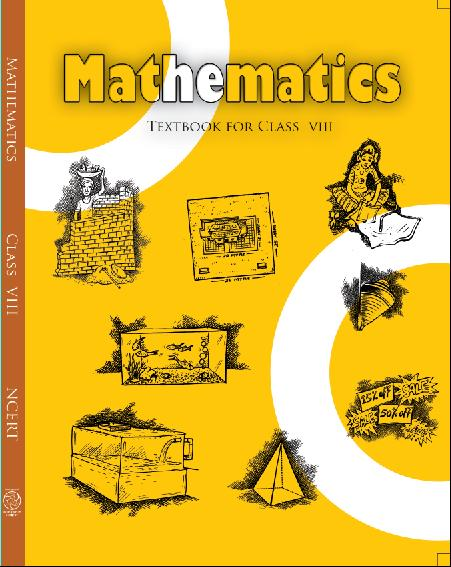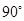1. /
2. CBSE
3. /
4. Class 08
5. /
6. Mathematics
7. /
8. NCERT Solutions for Class...

# NCERT Solutions for Class 8 Maths Exercise 3.4

NCERT solutions for Class 8 Maths Understanding Quadrilaterals## NCERT Solutions for Class 8 Maths Understanding Quadrilaterals

###### 1. State whether true or false:

(a) All rectangles are squares.

(b) All rhombuses are parallelograms.

(c) All squares are rhombuses and also rectangles.

(d) All squares are not parallelograms.

(e) All kites are rhombuses.

(f) All rhombuses are kites.

(g) All parallelograms are trapeziums.

(h) All squares are trapeziums.

Ans. (a) False. Since, squares have all sides are equal.

(b) True. Since, in rhombus, opposite angles are equal and diagonals intersect at mid-point.

(c) True. Since, squares have the same property of rhombus but not a rectangle.

(d) False. Since, all squares have the same property of parallelogram.

(e) False. Since, all kites do not have equal sides.

(f) True. Since, all rhombuses have equal sides and diagonals bisect each other.

(g) True. Since, trapezium has only two parallel sides.

(h) True. Since, all squares have also two parallel lines.

###### 2. Identify all the quadrilaterals that have:

(a) four sides of equal lengths.

(b) four right angles.

Ans. (a) Rhombus and square have sides of equal length.

(b) Square and rectangle have four right angles.

NCERT Solutions for Class 8 Maths Exercise 3.4

###### 3. Explain how a square is:

(b) a parallelogram

(c) a rhombus

(d) a rectangle

Ans. (i) A square is a quadrilateral, since it has four equal lengths of sides.

(ii) A square is a parallelogram, since it contains both pairs of opposite sides equal.

(iii) A square is already a rhombus. Since, it has four equal sides and diagonals bisect atto each other.

(iv) A square is a parallelogram, since having each adjacent angle a right angle and opposite sides are equal.

NCERT Solutions for Class 8 Maths Exercise 3.4

###### 4. Name the quadrilateral whose diagonals:

(i) bisect each other.

(ii) are perpendicular bisectors of each other.

(iii) are equal.

Ans. (i) If diagonals of a quadrilateral bisect each other then it is a rhombus, parallelogram,

rectangle or square.

(ii) If diagonals of a quadrilateral are perpendicular bisector of each other, then it is a rhombus or square.

(iii) If diagonals are equal, then it is a square or rectangle.

NCERT Solutions for Class 8 Maths Exercise 3.4

###### 5. Explain why a rectangle is a convex quadrilateral.

Ans. A rectangle is a convex quadrilateral since its vertex are raised and both of its diagonals lie in its interior.

## NCERT Solutions for Class 8 Maths Exercise 3.4

NCERT Solutions Class 8 Mathematics PDF (Download) Free from myCBSEguide app and myCBSEguide website. Ncert solution class 8 Mathematics includes text book solutions from Class 8 Maths Book . NCERT Solutions for CBSE Class 8 Maths have total 16 chapters. 8 Maths NCERT Solutions in PDF for free Download on our website. Ncert class 8 solutions PDF and Maths ncert class 8 PDF solutions with latest modifications and as per the latest CBSE syllabus are only available in myCBSEguide.

## CBSE app for Class 8

To download NCERT Solutions for class 8 Social Science, Computer Science, Home Science,Hindi ,English, Maths Science do check myCBSEguide app or website. myCBSEguide provides sample papers with solution, test papers for chapter-wise practice, NCERT solutions, NCERT Exemplar solutions, quick revision notes for ready reference, CBSE guess papers and CBSE important question papers. Sample Paper all are made available through the best app for CBSE students and myCBSEguide website.Test Generator

Create question paper PDF and online tests with your own name & logo in minutes.myCBSEguide

Question Bank, Mock Tests, Exam Papers, NCERT Solutions, Sample Papers, Notes

### 5 thoughts on “NCERT Solutions for Class 8 Maths Exercise 3.4”

1. It is to good.

2. I will understand all in maths

3. It’s very simple n rasy to understand…. keep it up!!

4. Where is ques 6???

5. The website is fraud and they say me to download as PDF then, I pressed it and they are saying to buy a book with Rs. 299. Don’t open the website